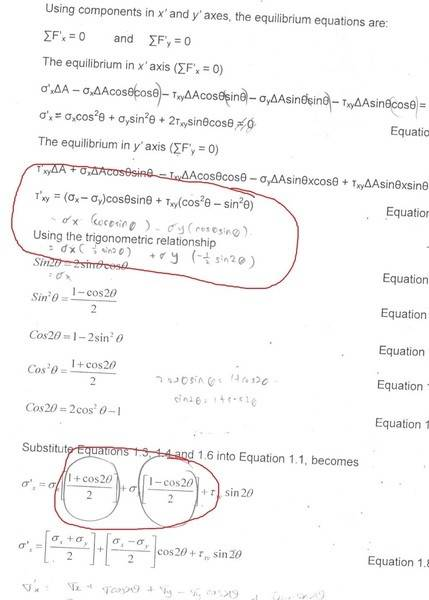# Trigonometric equation for stress

• foo9008

## Homework Statement

is the circled part wrong ?## The Attempt at a Solution

how could 0.5 sin2theta = ( 1 + cos2theta ) / 2 ?

foo9008 said:

## Homework Statement

is the circled part wrong ?## The Attempt at a Solution

how could 0.5 sin2theta = ( 1 + cos2theta ) / 2 ?
It's a trig identity. Look up "Half-Angle" formulas:

https://en.wikipedia.org/wiki/List_of_trigonometric_identities